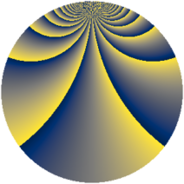# Properties

 Label 1140.2.wLevel $1140$ Weight $2$ Character orbit 1140.w Rep. character $\chi_{1140}(227,\cdot)$ Character field $\Q(\zeta_{4})$ Dimension $464$ Sturm bound $480$

# Related objects

## Defining parameters

 Level: $$N$$ $$=$$ $$1140 = 2^{2} \cdot 3 \cdot 5 \cdot 19$$ Weight: $$k$$ $$=$$ $$2$$ Character orbit: $$[\chi]$$ $$=$$ 1140.w (of order $$4$$ and degree $$2$$) Character conductor: $$\operatorname{cond}(\chi)$$ $$=$$ $$1140$$ Character field: $$\Q(i)$$ Sturm bound: $$480$$

## Dimensions

The following table gives the dimensions of various subspaces of $$M_{2}(1140, [\chi])$$.

Total New Old
Modular forms 496 496 0
Cusp forms 464 464 0
Eisenstein series 32 32 0

## Trace form

 $$464q - 8q^{6} + O(q^{10})$$ $$464q - 8q^{6} - 8q^{16} - 16q^{25} - 40q^{28} + 16q^{30} + 8q^{36} - 48q^{45} + 24q^{57} - 40q^{58} + 32q^{61} - 72q^{66} - 16q^{73} - 40q^{76} - 16q^{81} - 120q^{82} - 16q^{85} - 32q^{93} + 48q^{96} + O(q^{100})$$

## Decomposition of $$S_{2}^{\mathrm{new}}(1140, [\chi])$$ into newform subspaces

The newforms in this space have not yet been added to the LMFDB.